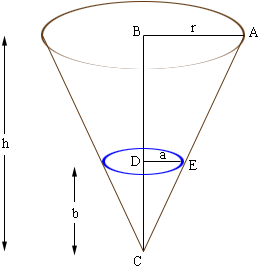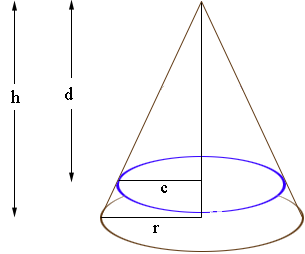Quandaries and Queries A vertically inverted cone( i.e. vertex down) has a radius 7 inches and height 24 inches. Water is filled to one third of its height .Find the ht of water when cone is turned upside down Hi, When I read this problem I saw a conical shaped paper cup, one-third full of water. When you turn it over all the water runs out so the height s zero. But this is probably not the answer you want. The important expression is that the volume of a cone is given by volume = 1/3 pir2h where r is the radius of the base and h is the height. You also have to use some information about similar triangles. Below is a sketch of one cone inside another as you have in your problem.The triangle ABC and EDC are similar so  r/h = a/b Using the volume expression, the volume of your cone is cone volume = 1/3 pi7 224 = 392 pi cubic inches. Use the similar triangle expression to find a and calculate the volume of the water in the cone by water volume = 1/3 pia 2b = 1/3 pia 28 cubic inches. The volume of air in the cone is (cone volume) - (water volume). Now turn the diagram upsidedown. Again you have similar triangles.This time  h/r = d/c Insert the values of h and r and solve for c in terms of d. Use the expression for the volume of a cone to write the volume of the air in terms of c and d. Use the volume for the air you found earlier and the expression for c in terms of d to form an equation in one variable d. Solve for d. Penny Go to Math Central